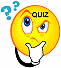Professor Freedman's
Elementary Algebra Homework Schedule
Fall 2020

Text: Beginning Algebra by Miller, O'Neill & Hyde, 5th Edition, McGrawHill, Inc.,2020

For Tutorials on Varied Topics Written by Fellow Students go to: http://www.mathpower.com/tutorial.htm
 DateAssigned Assignments Week of 9/2/20 Homework Assigned for week of 9/2/20 Watch the following Videos on Signed Numbers & Algebraic Expressions Introduction to Algebra -Exponents, Absolute Values & Order of Operations Addition & Subtraction of Signed Numbers Multiplication & Division of Signed Numbers Order of Operations on Signed Numbers Go to aleks.com: Do Assignments # 1,2,3Go to aleks.com Quiz #1 in Aleks on topics above Homework Tips (Week 1) Week of 9/7/20 Homework Assigned for week of 9/7/20 Watch the following Videos: Evaluating an Algebraic Expression Commutative, Associative & Distributive Properties Combining Like Terms Solving Linear Equations (Part 1) Go to aleks.com: Do Assignments # 4 & 5Go to aleks.com Quiz #2 in Aleks on topics above Week of 9/14/20 Homework Assigned for week of 9/14/20 Watch the following Videos: Solving Equations (Identities & Contradictions) Literal Equations Go to aleks.com Assignment #6 Solving Equations (Multiple Steps), Assignment #7 Word Problems, Identity & Contradiction Equations &Assignment #8 Literal EquationsGo to aleks.com Quiz #3 on Equations, Word Problems, Literal Equations Week of 9/21/20 Homework Assigned for week of 9/21/20 Watch the following Videos on Inequalities : Graphing Inequalities Solving Inequalities Watch the following Videos on Graphing : Identifying Solutions of 2 Variable Equations (Intro to Graphing) Plotting Points Graphing Straight Lines Slope of a Line Go to aleks.com Assignment #9 (Inequalities) & Assignment #10 (Graphing Styraight Line)s & Assignment #11 (Slope of a Line)Go to aleks.com Quiz #4 on Inequalities & Graphing Week of 9/28/20 MAJOR TEST on Chapters 1,2,3 Study: Do the Practice Test and then check your answers. PRACTICE TEST (Chapters,1,2,3) Week of 10/5/20 Homework Assigned for week of 10/5/20 Watch the following Videos on Systems of Equations : Solving Systems of Equations by Addition (Part 1) Solving Systems of Equations by Addition (Part 2) Solving Systems of Equations by Substitution Go to aleks.com Assignment #12 (Solving Systems of Equations by Graphing and by Addition) & Assignment #13 (Solving Word Prpoblems using Systems of Equations and Solving Systems of Equations by Substitution)Go to aleks.com Quiz #5 on Systems of Equations Week of 10/12/20 Homework Assigned for week of 10/12/20 Watch the following Videos on Exponent Rules : Multiplying & Dividing Variables Zero Exponents Negative Exponents Watch the following Videos on Adding & Subtracting Polynomials : Writing a Polynomial in Standard Form Addition & Subtraction of Polynomials Watch the following Videos on Multiplying Polynomials : Multiplication of Polynomials Part 1 Multipliction of Polynomials FOIL Part 2 Polynomial Special Products Go to aleks.com Assignment #14 (Exponent Rules) & Assignment #15 (Addition & Subtraction of Polynomials) & Assignment #16 ( Multiplication of Polynomials) Week of 10/19/20 Go to aleks.com Homework Assignment #17 (Practice Midterm Exam,) Due 10/22 MIDTERM EXAM Due 10/25 Week of 10/26/20 Homework Assigned for week of 10/26/20 Watch the following Videos: Polynomial Division Factoring Polynomials                   Go to aleks.com Assignment #18 (Division of Polynomials) , Assignment #19 (Factoring Greatest Common Factor) , Assignment #20 ( Factoring Difference of Two Squares), and Assignment #21 (Factoring Trinomials with a Leading Coefficient of One) Homework Tips (Factoring Difference of 2 Squares & Trinomials) Week of 11/2/20 Homework Assigned for week of 11/2/20 Watch the following Videos: Go to aleks.com Assignment #22 (Factoring Trinomials with Leading Coefficient Other than One - Trial and Error) , Assignment #23 (Solving Quadratic Equations), Assignment #24 Mixed FactoringGo to aleks.com Quiz #6 on Factoring Greatest Common Factor, Difference of Two Squares , Trinomials and Solving Quadratic Equations Homework Tips (Factoring Trinomials) Week of 11/9/20 Homework Assigned for week of 11/9/20 Watch the following Videos: Multiplying & Dividing Algebraic Fractions with Factoring Go to aleks.com Assignment #25 (Simplifying Algebraic Fractions) , Assignment #26 (Multiplying and Dividing Algebraic Fractions)Go to aleks.com Quiz #7 on Simplifying Algebraic Fractions and Multiplying and Dividing Algebraic Fractions Week of 11/16/20 Homework Assigned for week of 11/16/20 Watch the following Videos: Addition & Subtraction of Rational Expressions with Different Denominators (Part 1) Addition & Subtraction of Rational Expressions with Different Denominators (Part 2) Solving Rational Equations (Equations with Algebraic Fractions) Go to aleks.com Assignment #27 (Adding & Subtracting Algebraic Fractions with Same Denominator) , Assignment #28 (Adding & Subtracting Algebraic Fractions with Different Denominators) , Assignment 29 (Solving Rational Equations), Assignment #30 (Practice Test 3 on Factoring & Algebraic Fractions- Study Guide) Week of 11/23/20 Homework Assigned for week of 11/23/20 Go to aleks.com MAJOR TEST ON FACTORING & ALGEBRAIC FRACTIONS in Aleks.com due 11/30 Week of 11/30/20 Homework Assigned for week of 11/30/20 Watch the following Videos: Simplifying Radicals (Numbers) Simplifying Radicals with Variables Go to aleks.com Assignment #31 (Simplifying Radicals) & Assignment #32 Practice Final Exam - Study Guide Week of 12/7/20 Go to aleks.com FINAL EXAM